# NCERT Exemplar Solutions for Class 10 Maths Chapter 7 - Coordinate Geometry

## NCERT Exemplar Solutions Class 10 Maths Chapter 7 – Free PDF Download

NCERT Exemplar Class 10 Maths Chapter 7 Coordinate Geometry, provided here for students to prepare for CBSE exams in PDF format. These exemplars problems and solutions are designed by experts with respect to the CBSE Syllabus(2021-2022). The exemplar can further be used as an effective study tool and will seemingly help students develop better Math fluency and tackle even the difficult questions that can be asked in the CBSE first and second term exam.

In Chapter 7 of NCERT Exemplar Class 10 Solutions students will be introduced to different formulas like distance and section formula and they will have to understand its application properly. The chapter also deals with topics like the area of a triangle and students have to work on problems based on them. While this is the main objective of the chapter, which basically helps students focus more on applying the concepts of coordinate geometry to the real-world scenarios.

This chapter covers the following topics given below:

• Finding the distance between two points in two coordinates
• Section formula and finding the coordinates of the point which divides a line segment
• Finding the area of triangles with the help of coordinate points
• Finding the mid-point of the line segment joining the coordinate points

## Download PDF of NCERT Exemplar Solutions for Class 10 Maths Chapter 7 Coordinate Geometry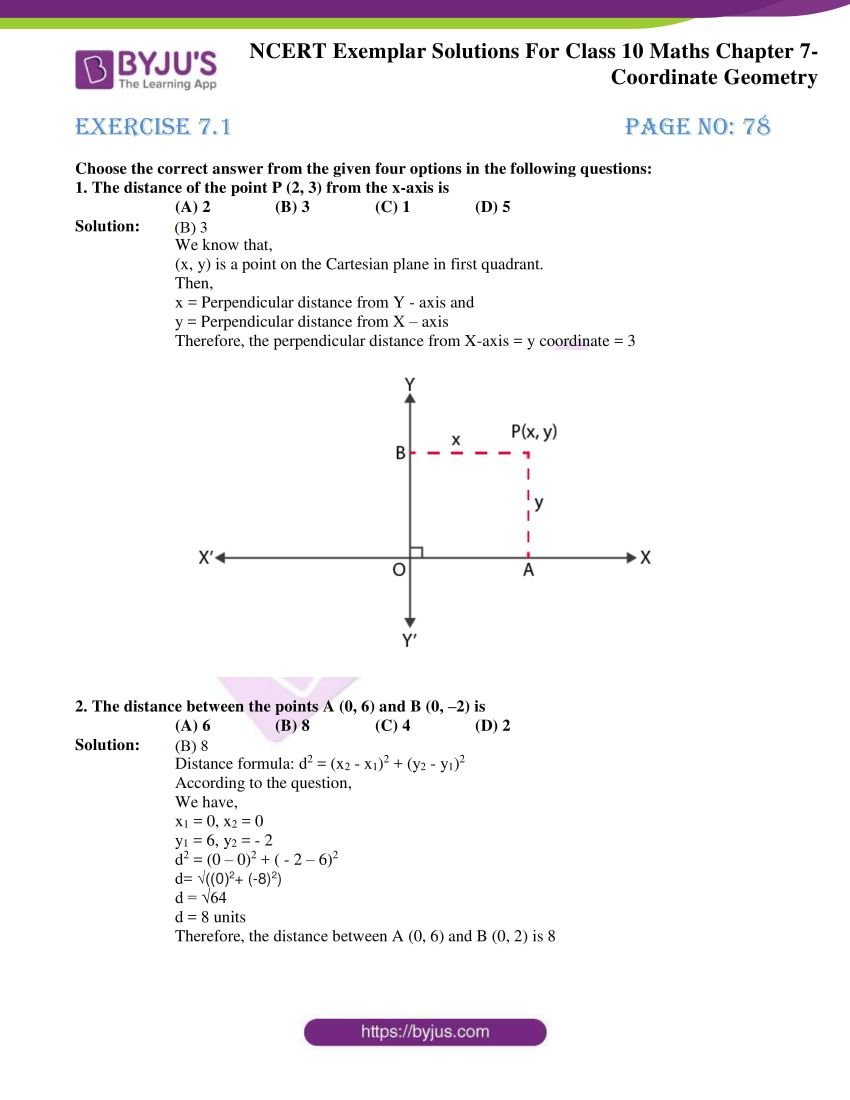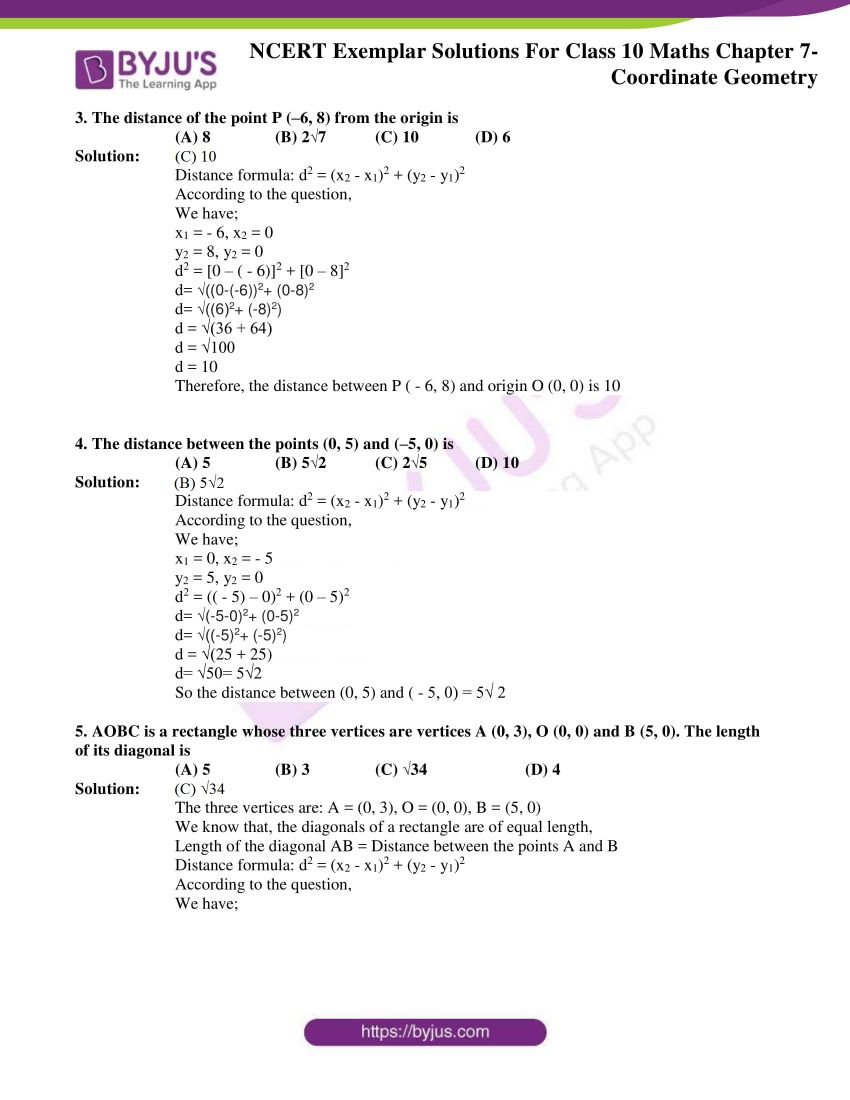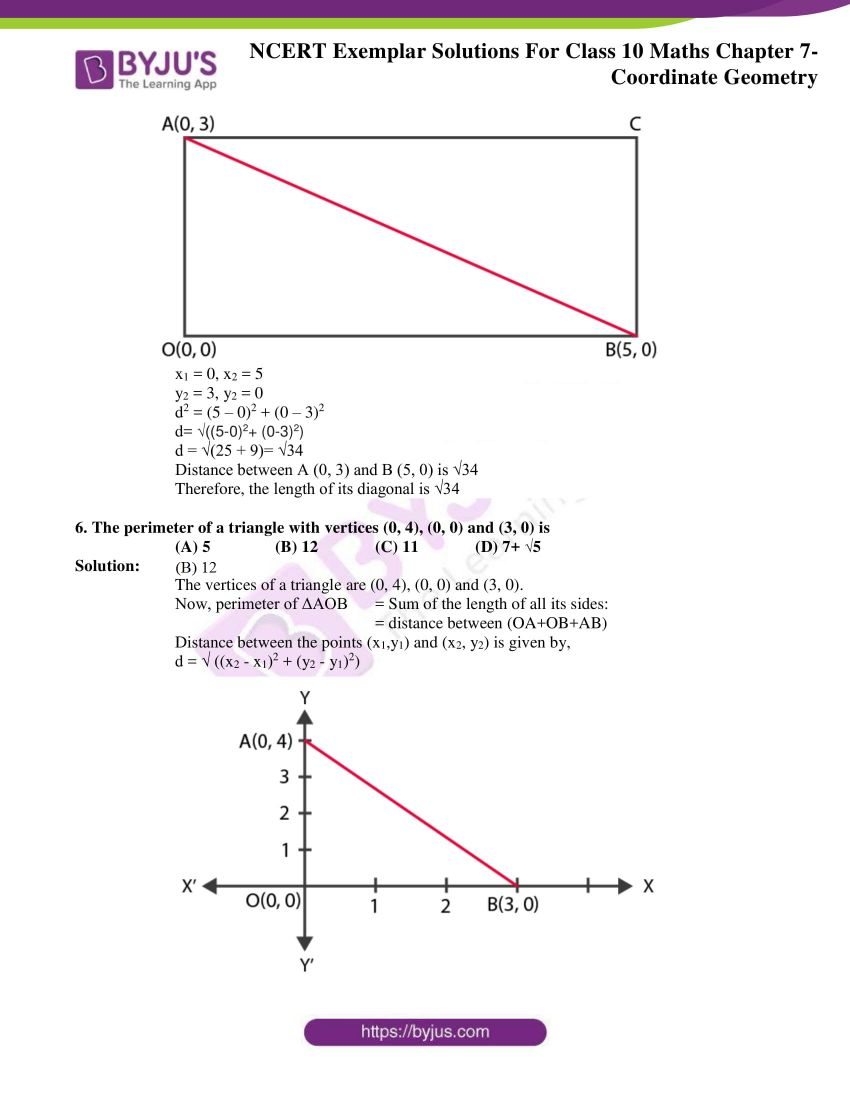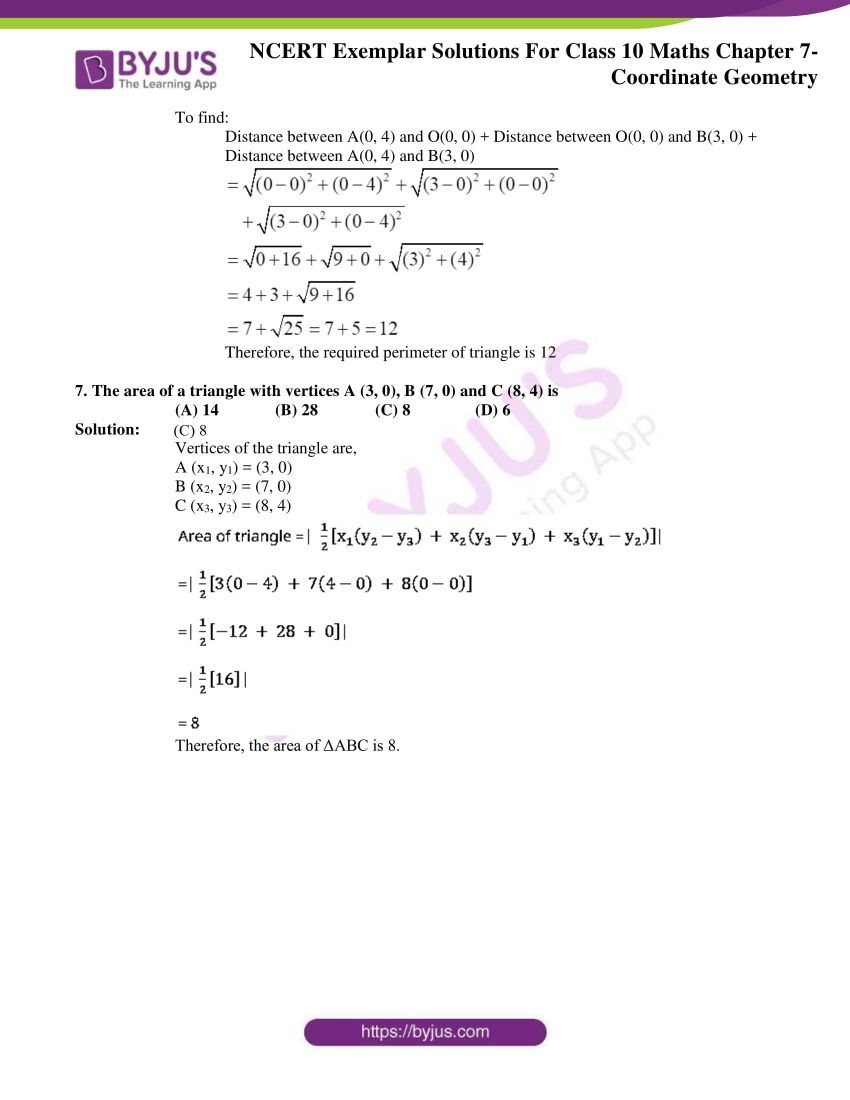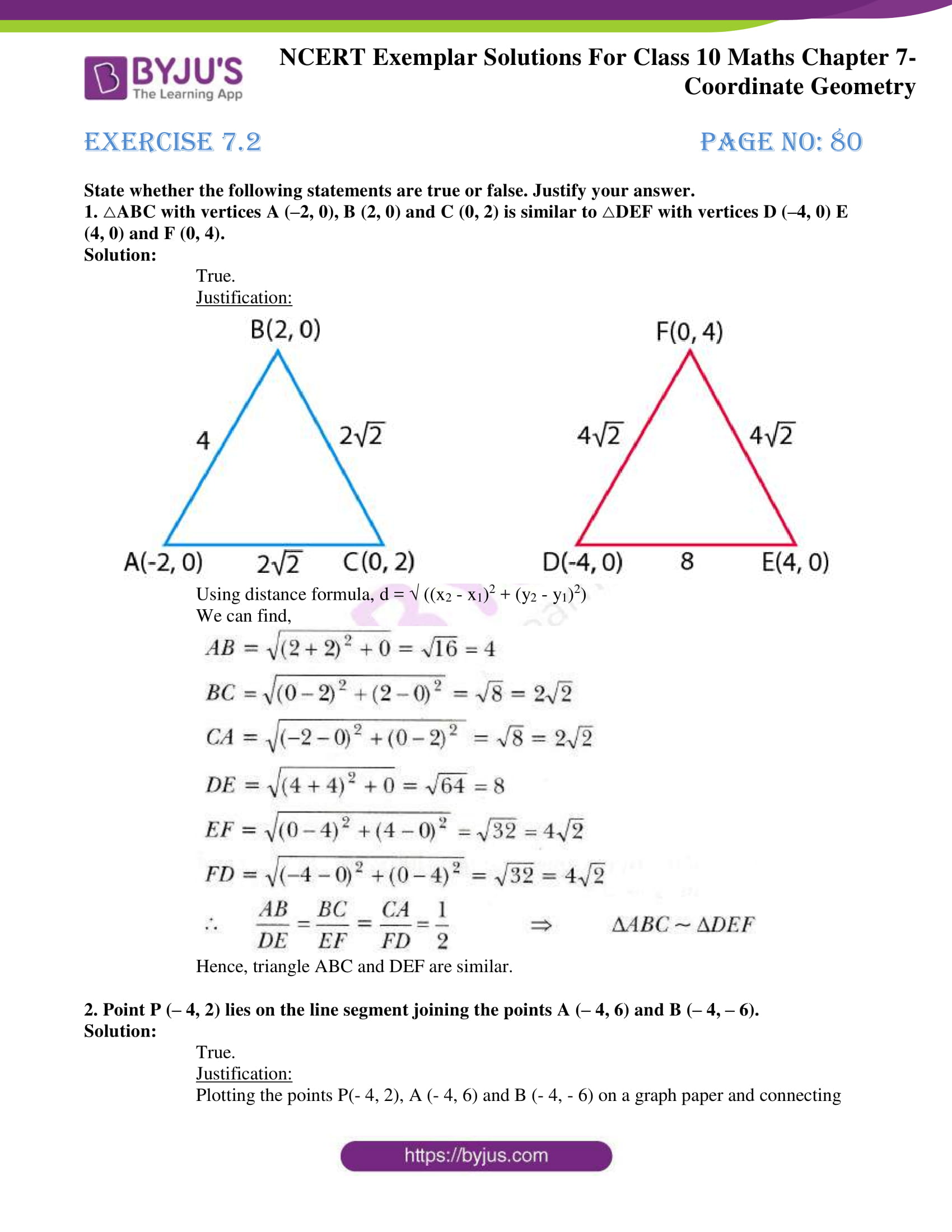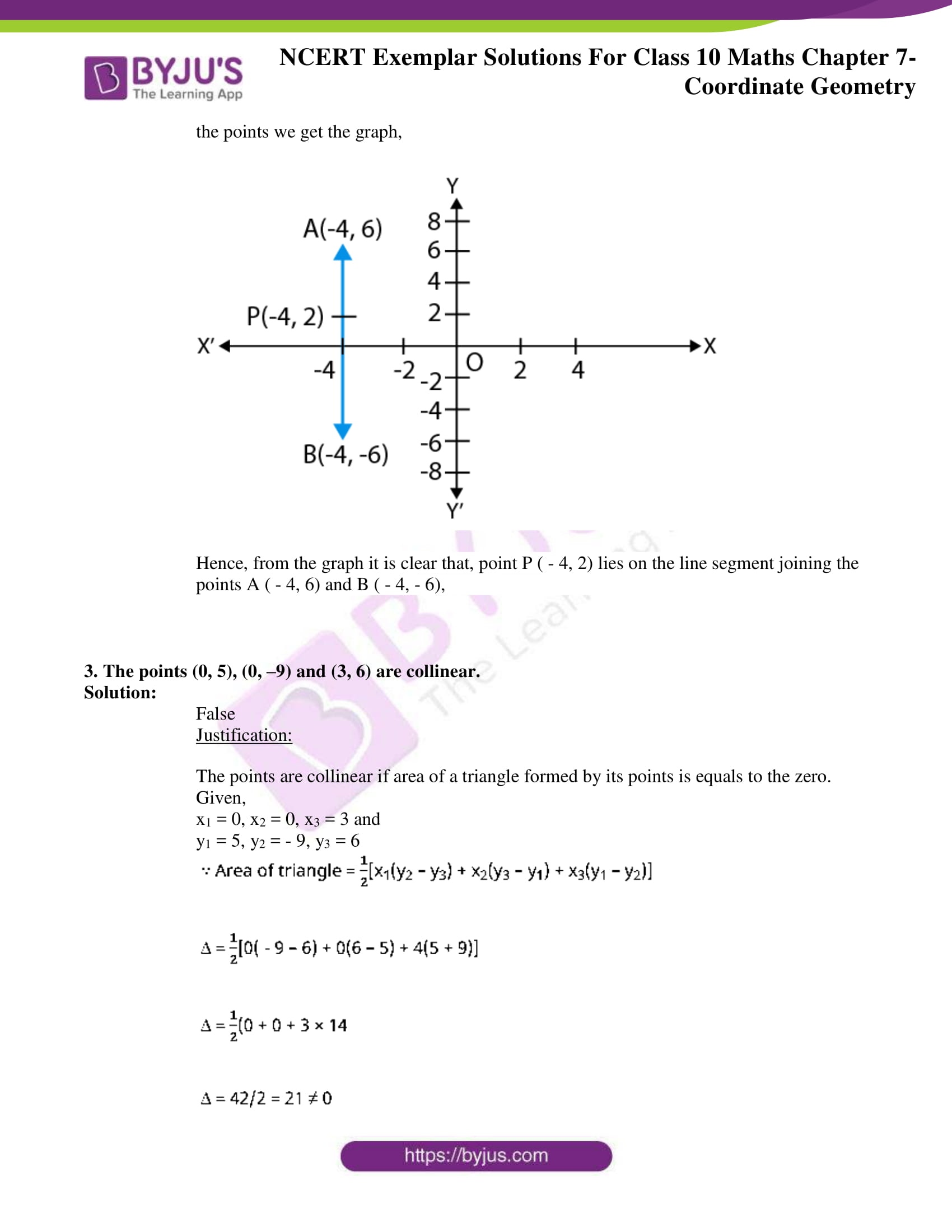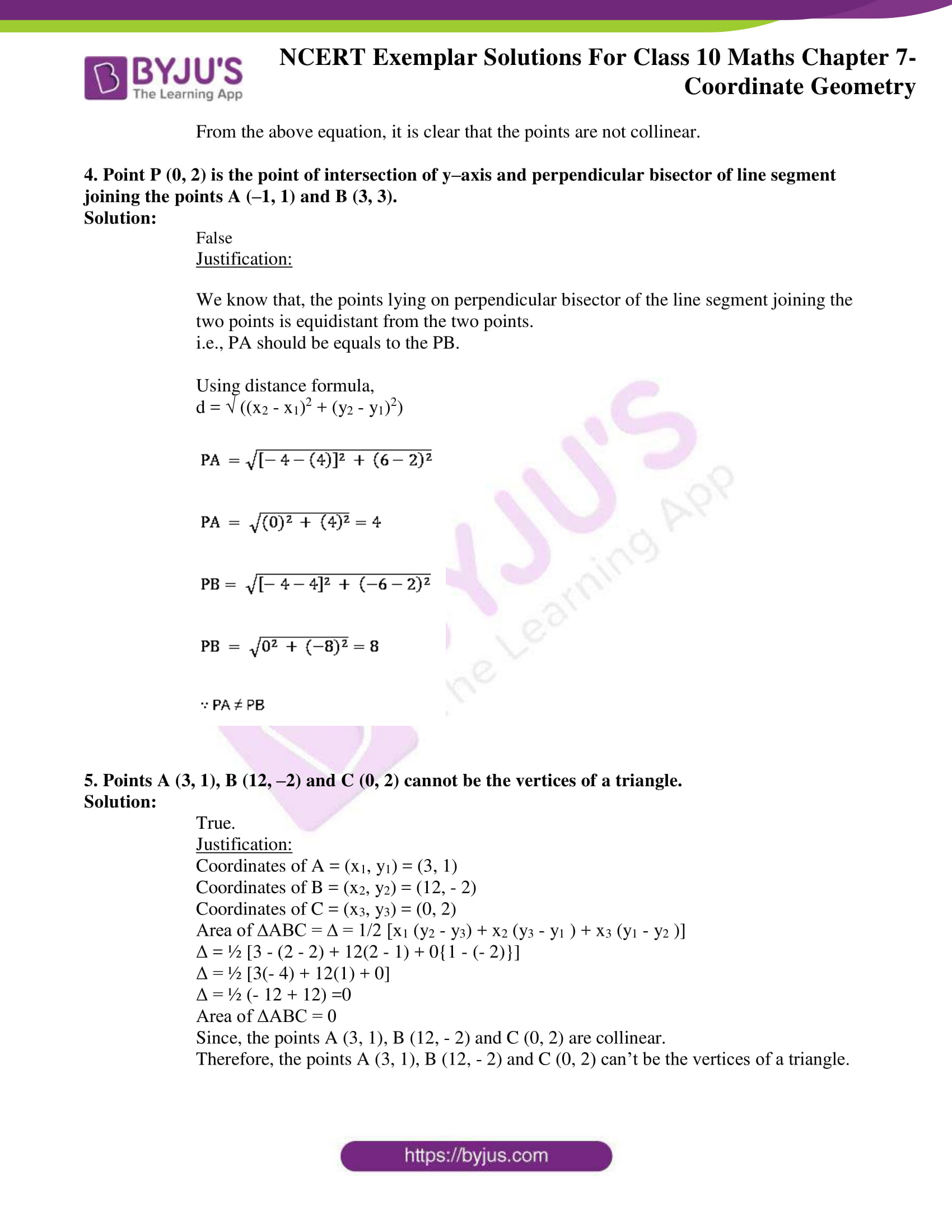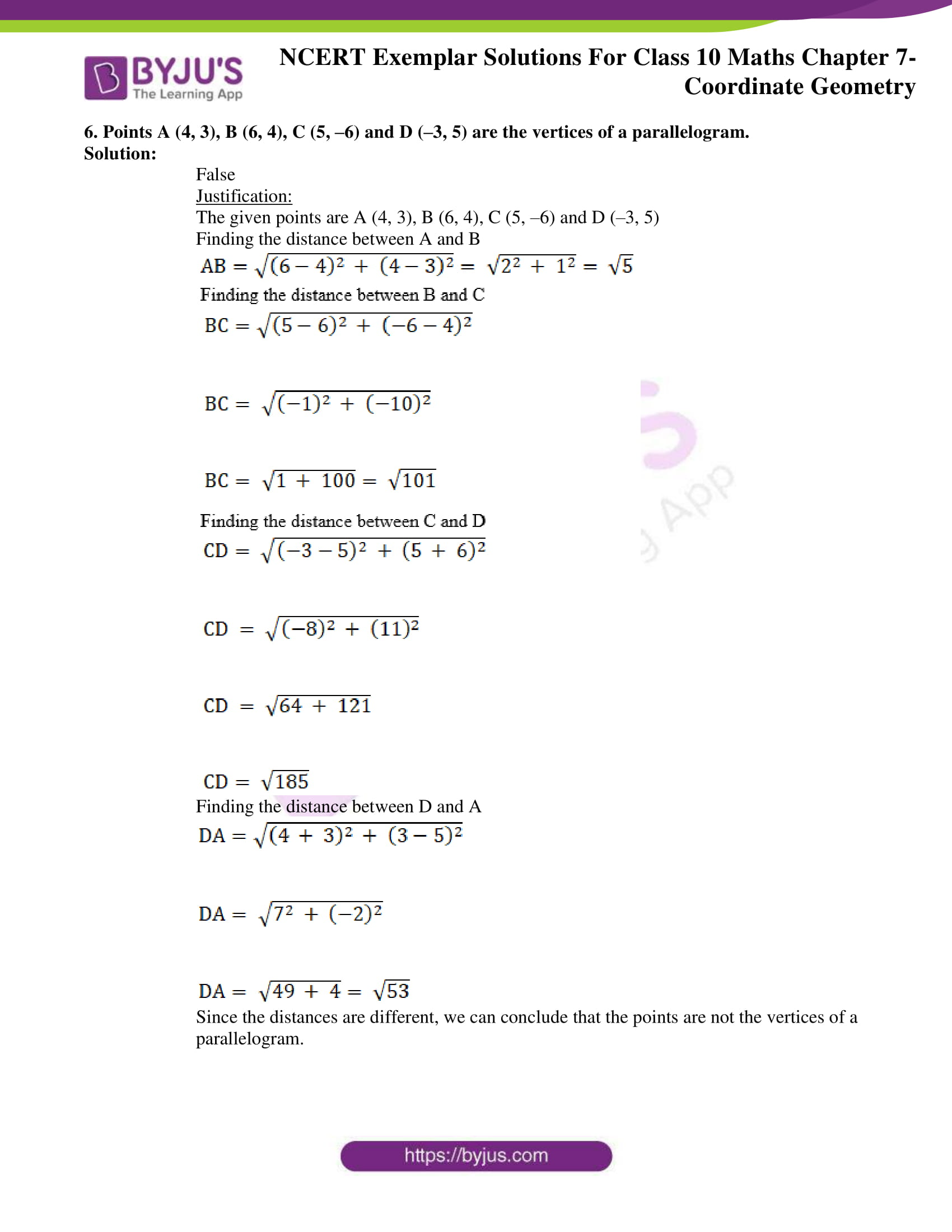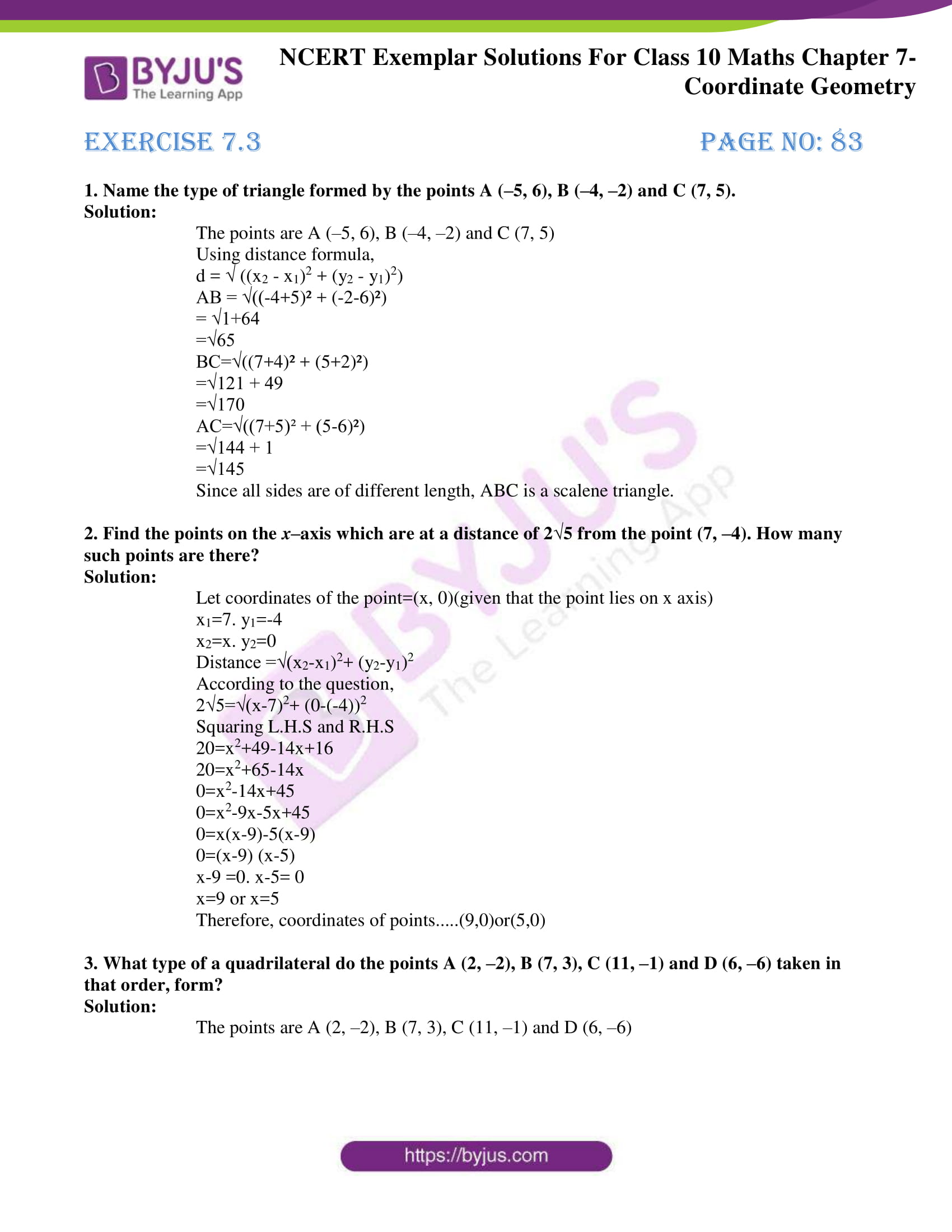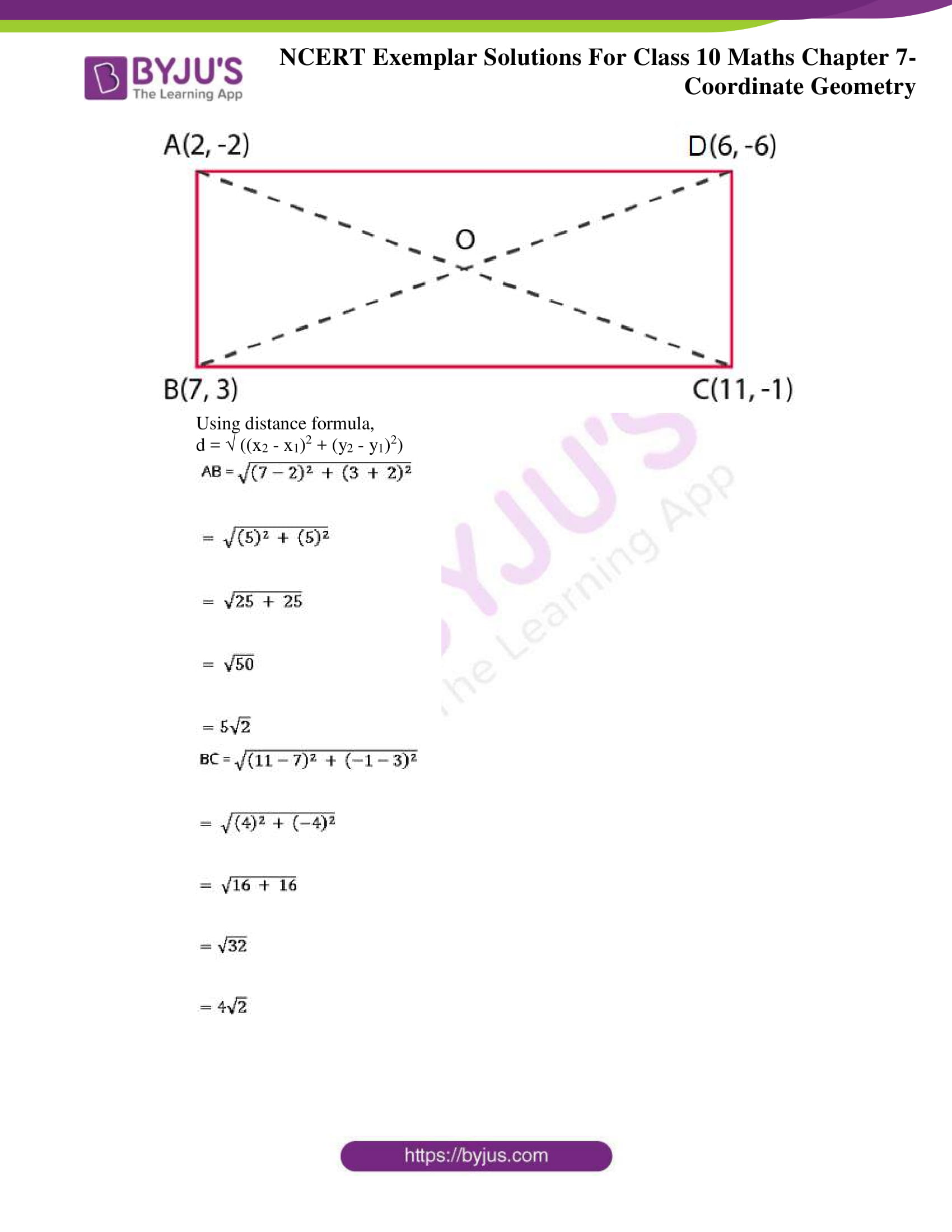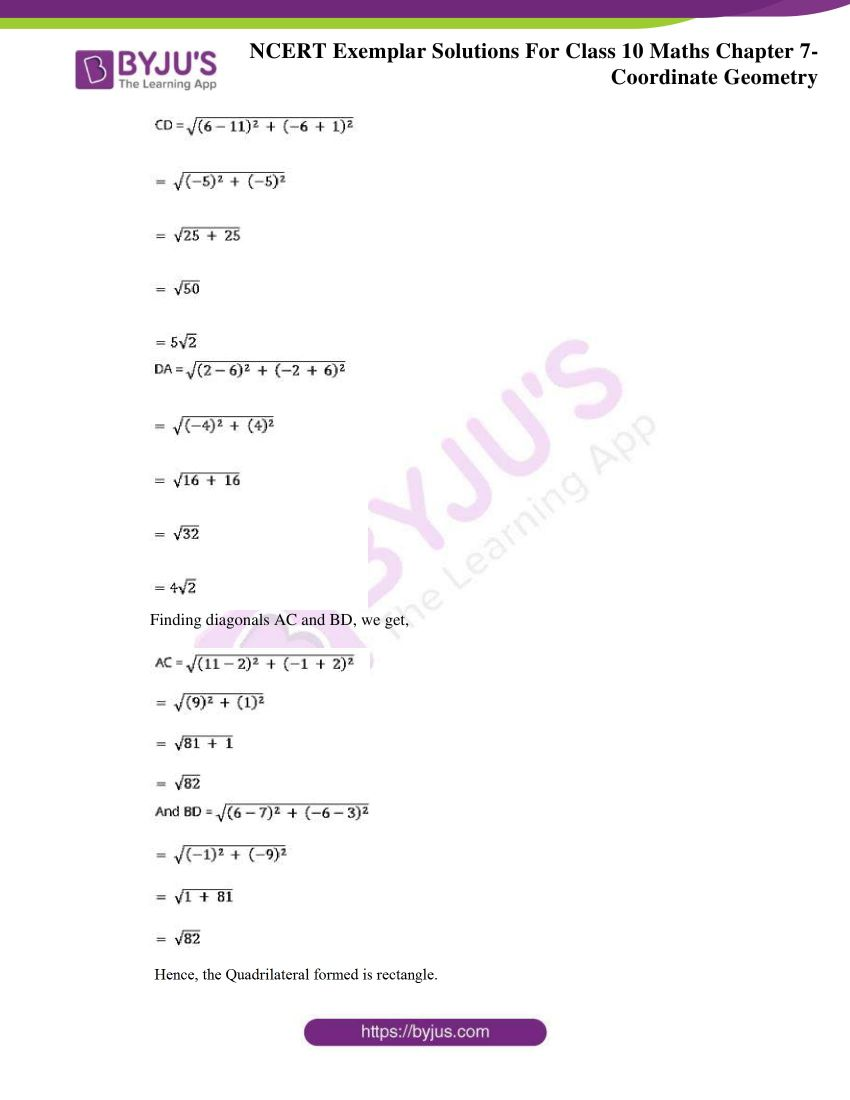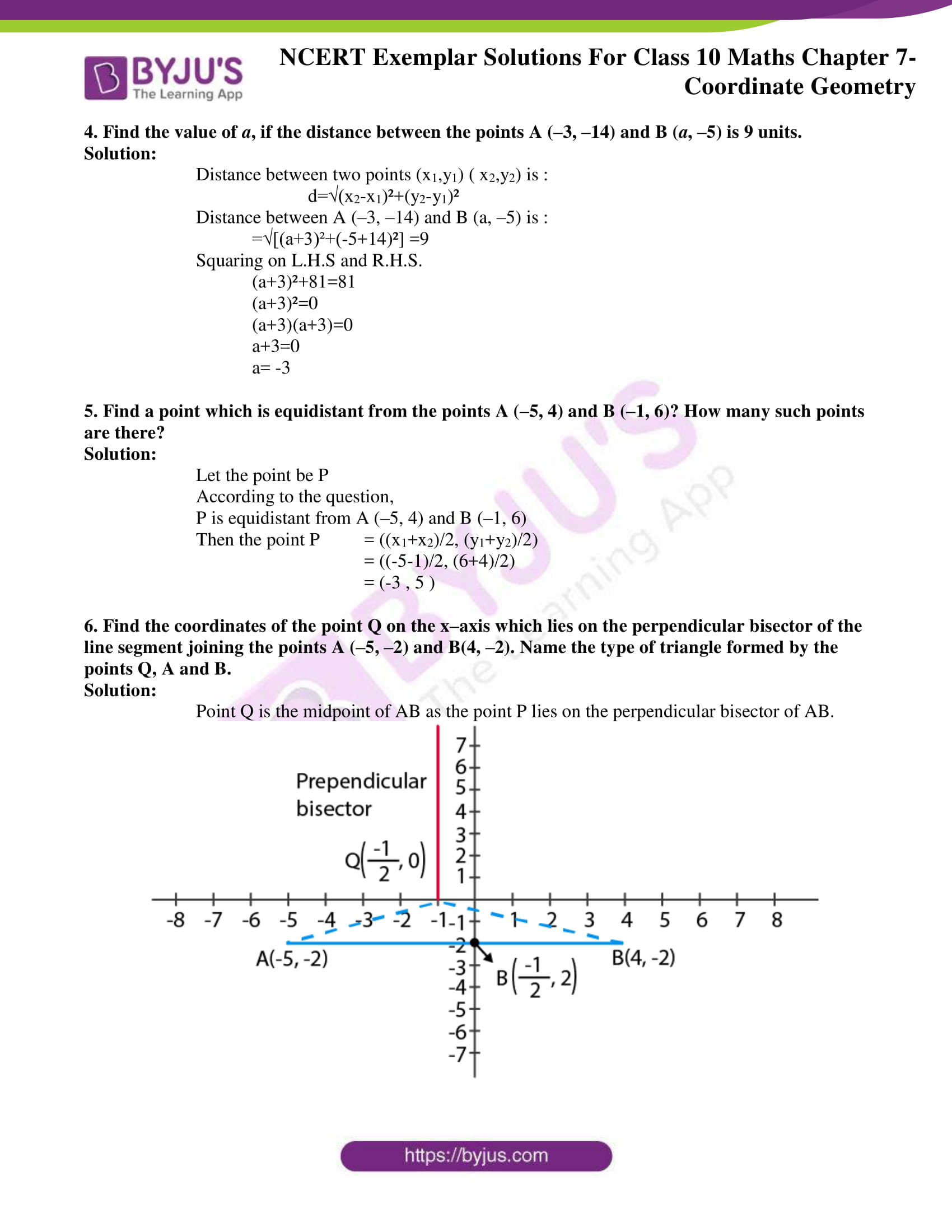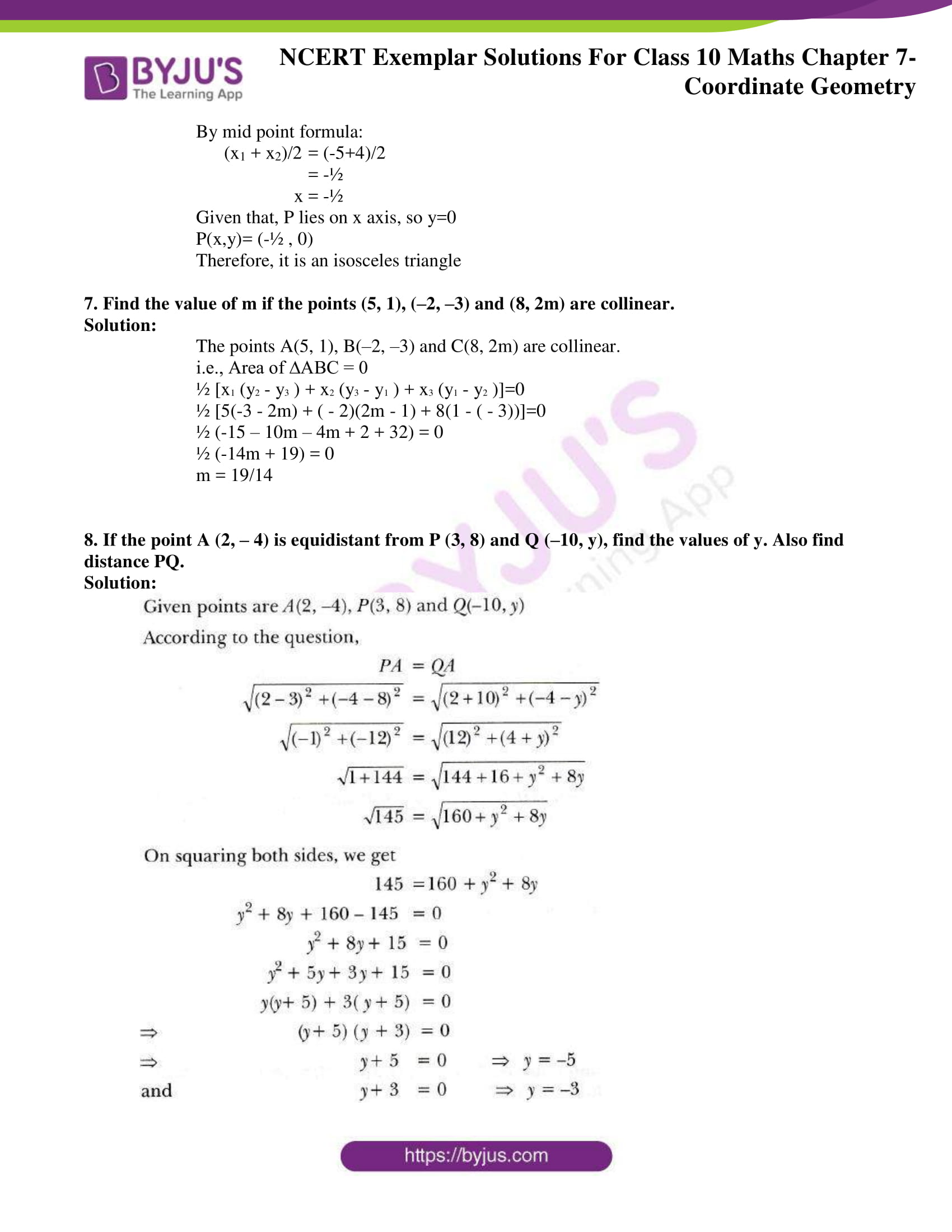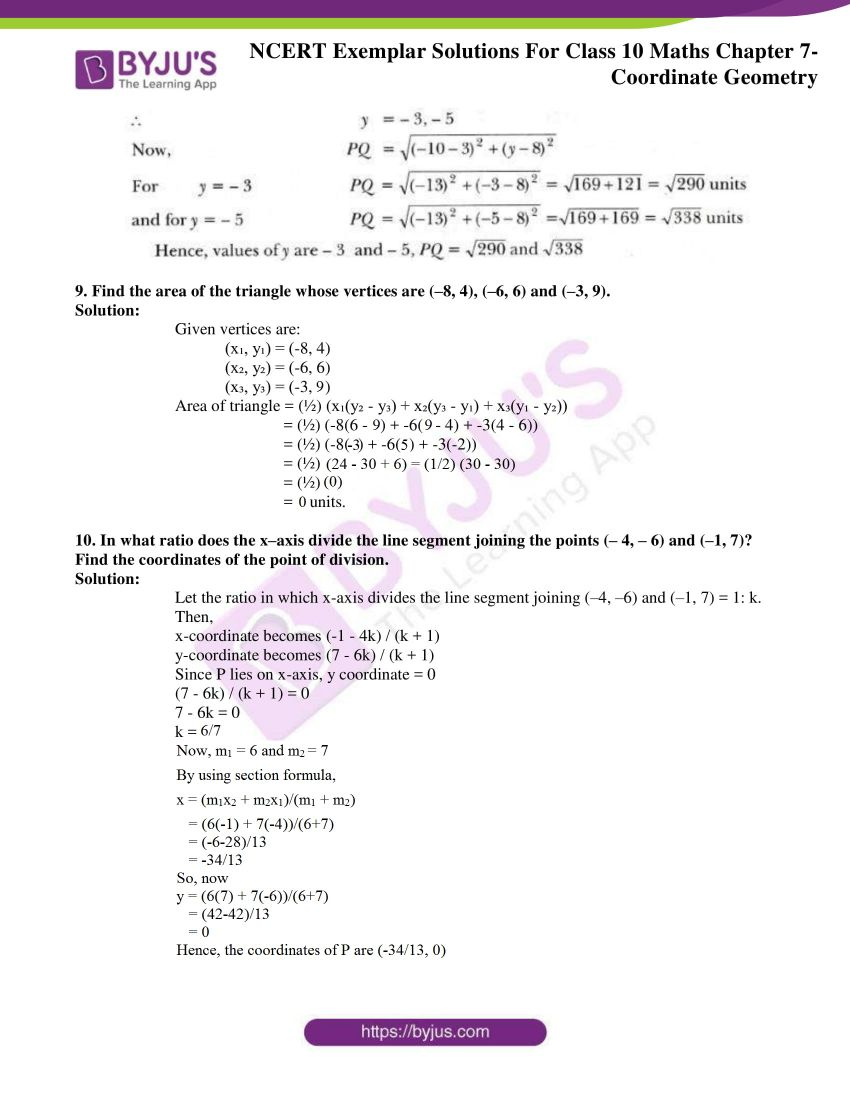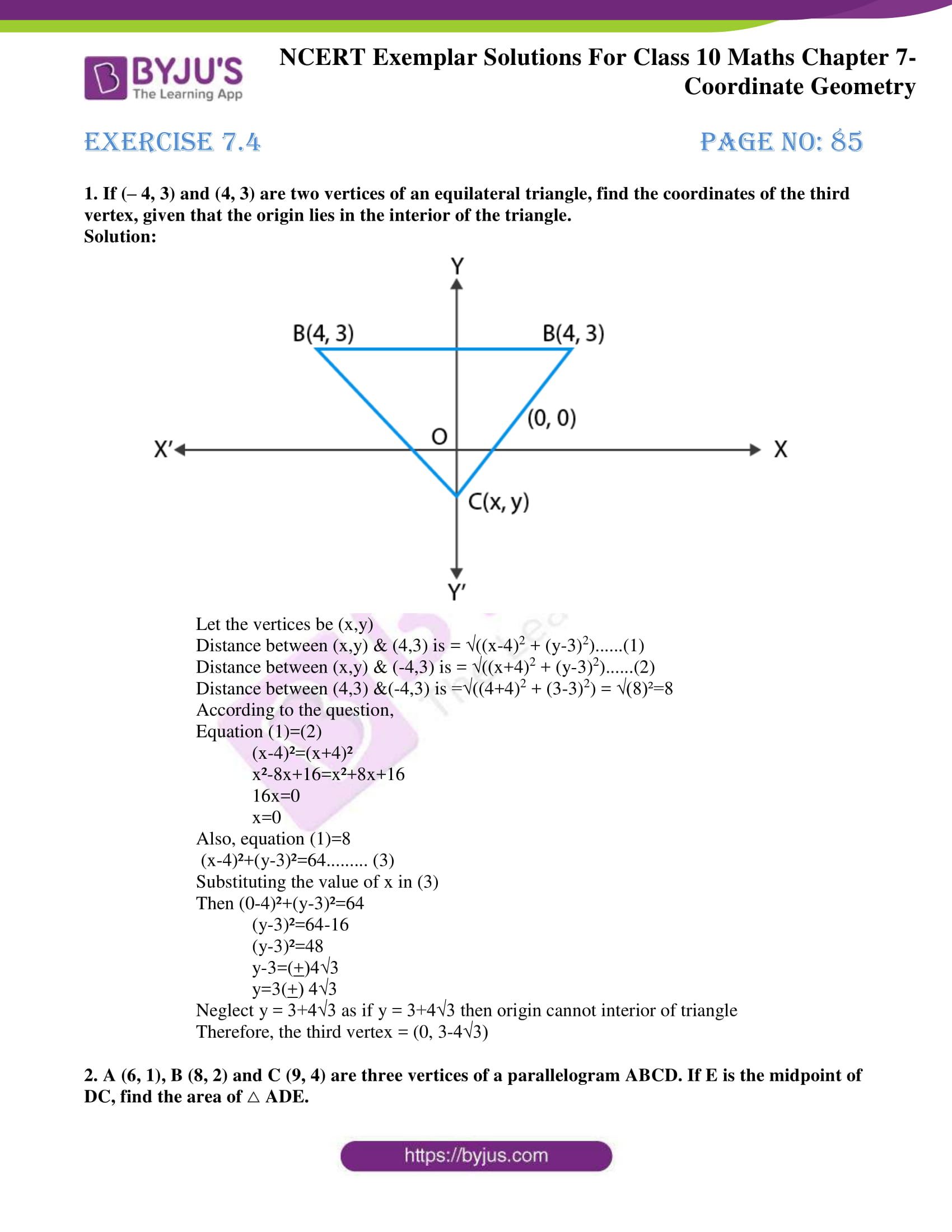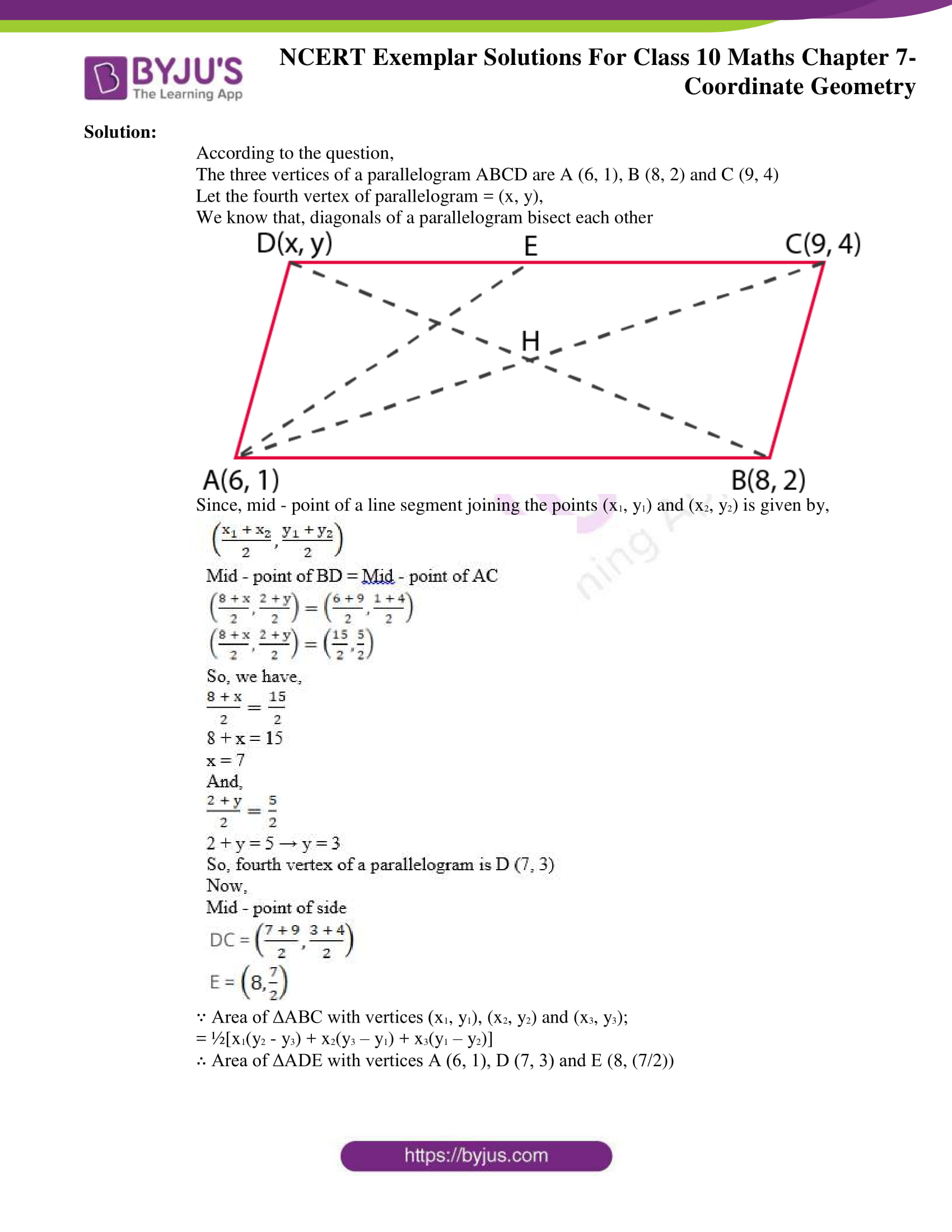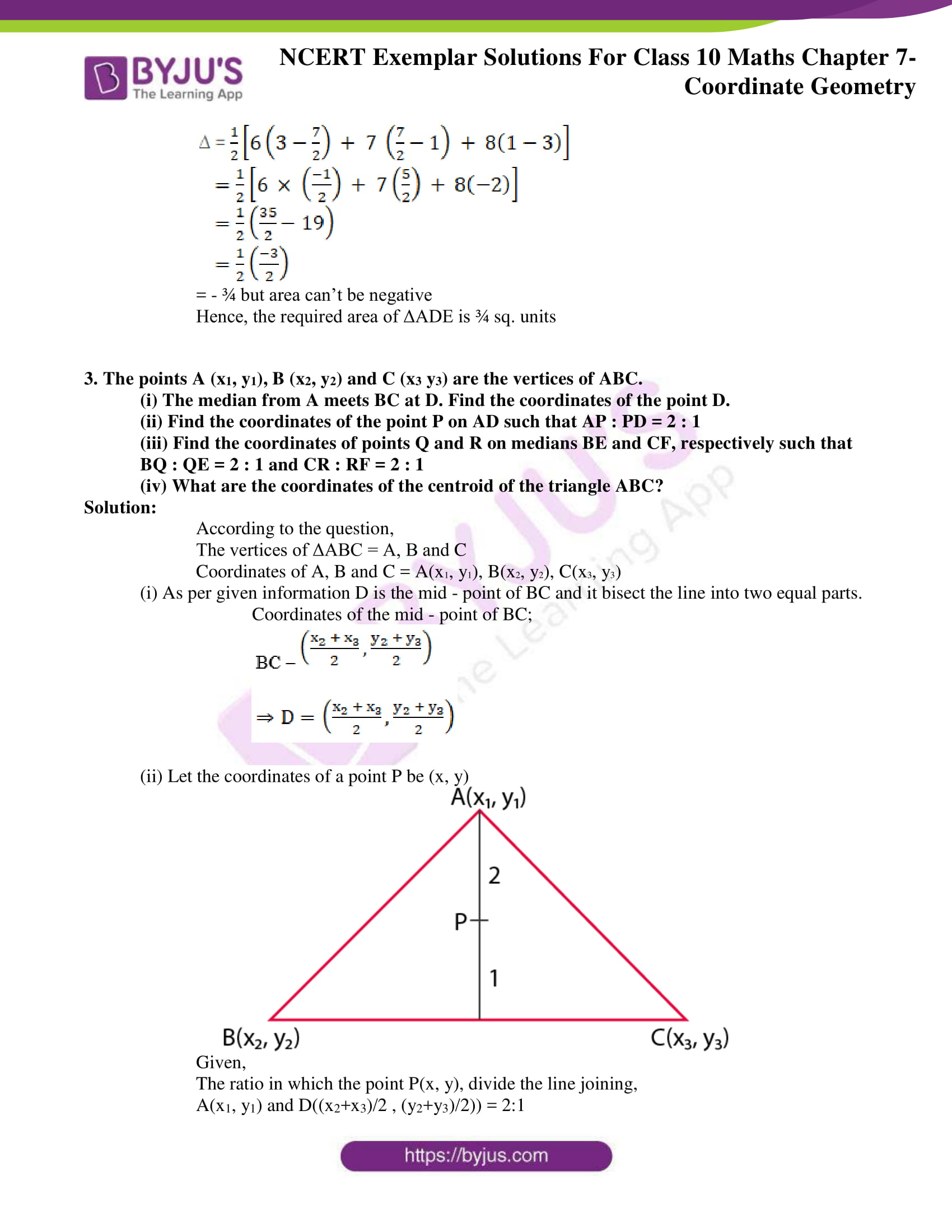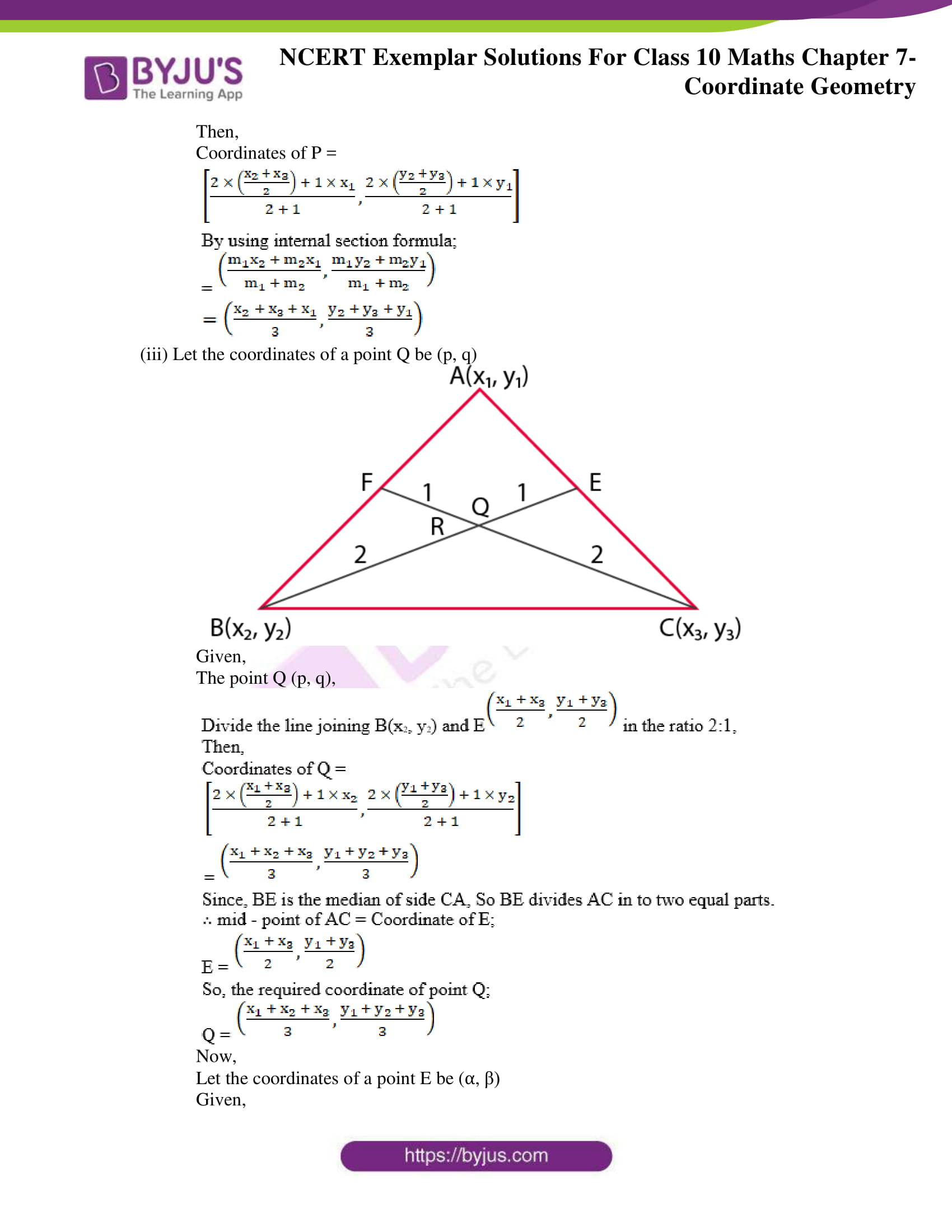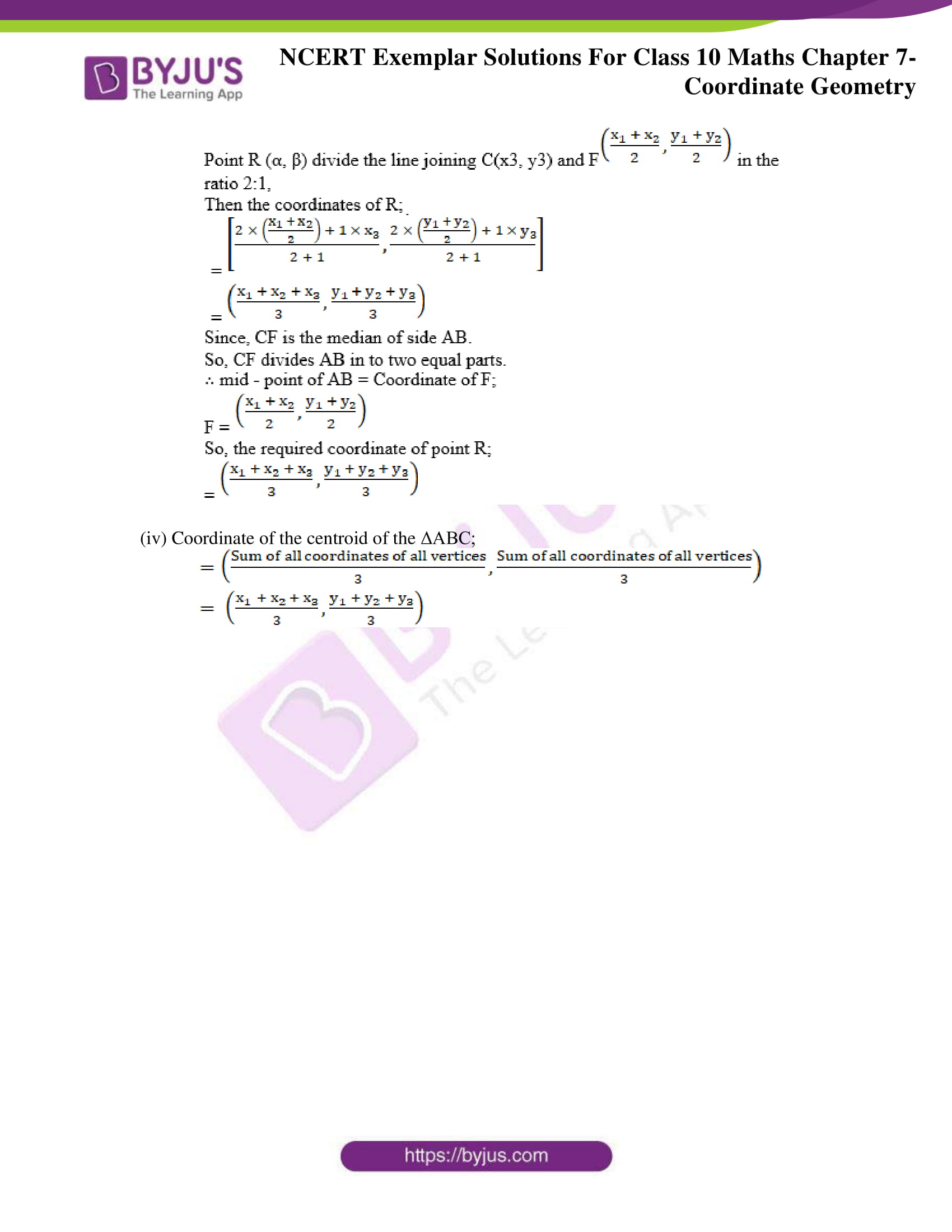## Exercise 7.1 Page No: 78

Choose the correct answer from the given four options in the following questions:

1. The distance of the point P (2, 3) from the x-axis is

(A) 2 (B) 3 (C) 1 (D) 5

Solution:

(B) 3

We know that,

(x, y) is a point on the Cartesian plane in first quadrant.

Then,

x = Perpendicular distance from Y – axis and

y = Perpendicular distance from X – axis

Therefore, the perpendicular distance from X-axis = y coordinate = 3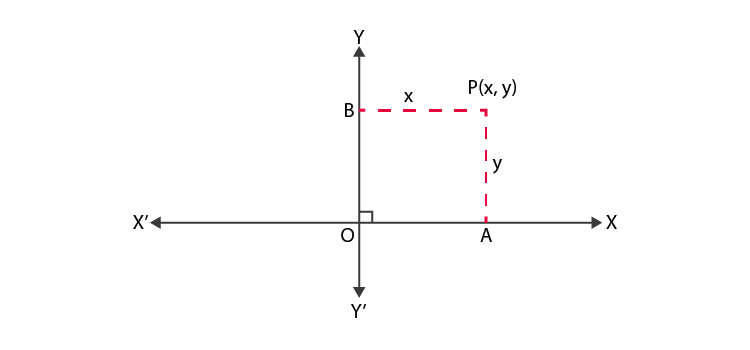2. The distance between the points A (0, 6) and B (0, –2) is

(A) 6 (B) 8 (C) 4 (D) 2

Solution:

(B) 8

Distance formula: d2 = (x2 – x1)2 + (y2 – y1)2

According to the question,

We have,

x1 = 0, x2 = 0

y1 = 6, y2 = – 2

d2 = (0 – 0)2 + ( – 2 – 6)2

d= √((0)2+ (-8)2)

d = √64

d = 8 units

Therefore, the distance between A (0, 6) and B (0, 2) is 8

3. The distance of the point P (–6, 8) from the origin is

(A) 8 (B) 27 (C) 10 (D) 6

Solution:

(C) 10

Distance formula: d2 = (x2 – x1)2 + (y2 – y1)2

According to the question,

We have;

x1 = – 6, x2 = 0

y2 = 8, y2 = 0

d2 = [0 – ( – 6)]2 + [0 – 8]2

d= √((0-(-6))2+ (0-8)2

d= √((6)2+ (-8)2)

d = √(36 + 64)

d = √100

d = 10

Therefore, the distance between P ( – 6, 8) and origin O (0, 0) is 10

4. The distance between the points (0, 5) and (–5, 0) is

(A) 5 (B) 52 (C) 25 (D) 10

Solution:

(B) 5√ 2

Distance formula: d2 = (x2 – x1)2 + (y2 – y1)2

According to the question,

We have;

x1 = 0, x2 = – 5

y2 = 5, y2 = 0

d2 = (( – 5) – 0)2 + (0 – 5)2

d= √(-5-0)2+ (0-5)2

d= √((-5)2+ (-5)2)

d = √(25 + 25)

d= √50= 5√2

So the distance between (0, 5) and ( – 5, 0) = 5√ 2

5. AOBC is a rectangle whose three vertices are vertices A (0, 3), O (0, 0) and B (5, 0). The length of its diagonal is

(A) 5 (B) 3 (C) 34 (D) 4

Solution:

(C) √34

The three vertices are: A = (0, 3), O = (0, 0), B = (5, 0)

We know that, the diagonals of a rectangle are of equal length,

Length of the diagonal AB = Distance between the points A and B

Distance formula: d2 = (x2 – x1)2 + (y2 – y1)2

According to the question,

We have;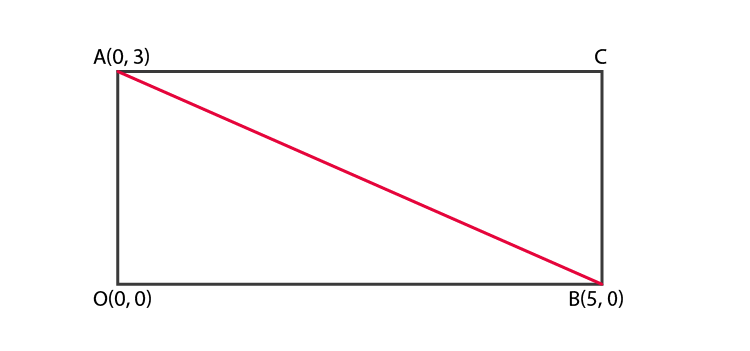x1 = 0, x2 = 5

y2 = 3, y2 = 0

d2 = (5 – 0)2 + (0 – 3)2

d= √((5-0)2+ (0-3)2)

d = √(25 + 9)= √34

Distance between A (0, 3) and B (5, 0) is √34

Therefore, the length of its diagonal is √34

6. The perimeter of a triangle with vertices (0, 4), (0, 0) and (3, 0) is

(A) 5 (B) 12 (C) 11 (D) 7+5

Solution:

(B) 12

The vertices of a triangle are (0, 4), (0, 0) and (3, 0).

Now, perimeter of ΔAOB = Sum of the length of all its sides:

= distance between (OA+OB+AB)

Distance between the points (x1,y1) and (x2, y2) is given by,

d = √ ((x2 – x1)2 + (y2 – y1)2)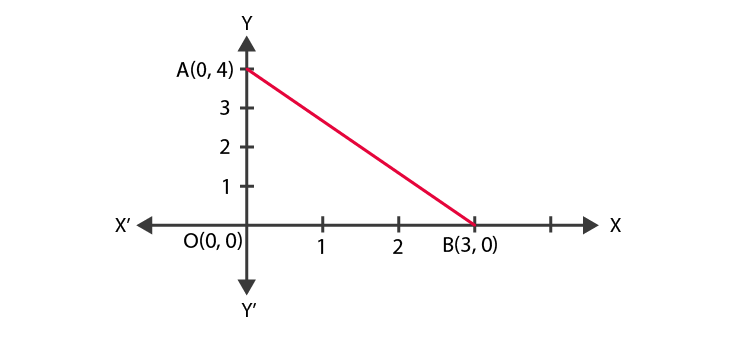To find:

Distance between A(0, 4) and O(0, 0) + Distance between O(0, 0) and B(3, 0) + Distance between A(0, 4) and B(3, 0)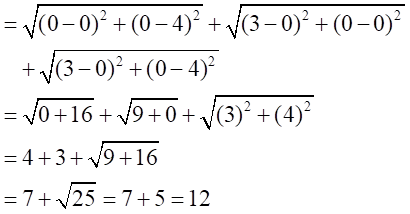Therefore, the required perimeter of triangle is 12

7. The area of a triangle with vertices A (3, 0), B (7, 0) and C (8, 4) is

(A) 14 (B) 28 (C) 8 (D) 6

Solution:

(C) 8

Vertices of the triangle are,

A (x1, y1) = (3, 0)

B (x2, y2) = (7, 0)

C (x3, y3) = (8, 4)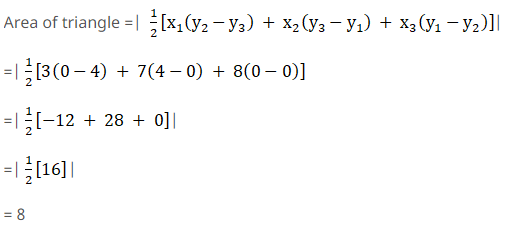Therefore, the area of ΔABC is 8.

## Exercise 7.2 Page No: 80

State whether the following statements are true or false. Justify your answer.

1. ABC with vertices A (–2, 0), B (2, 0) and C (0, 2) is similar to DEF with vertices D (–4, 0) E (4, 0) and F (0, 4).

Solution:

True.

Justification: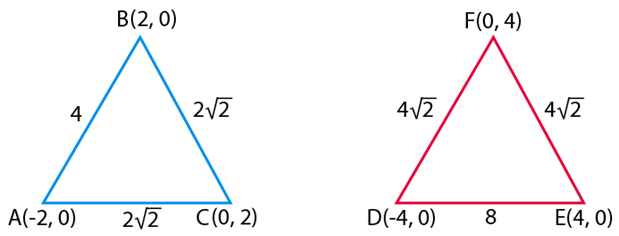Using distance formula, d = √ ((x2 – x1)2 + (y2 – y1)2)

We can find,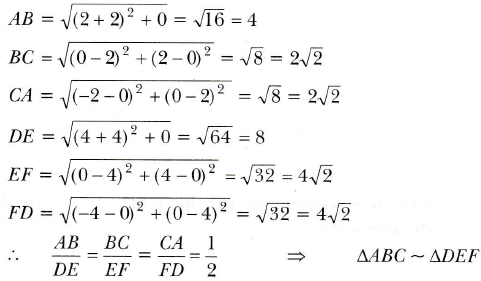Hence, triangle ABC and DEF are similar.

2. Point P (– 4, 2) lies on the line segment joining the points A (– 4, 6) and B (– 4, – 6).

Solution:

True.

Justification:

Plotting the points P(- 4, 2), A (- 4, 6) and B (- 4, – 6) on a graph paper and connecting the points we get the graph,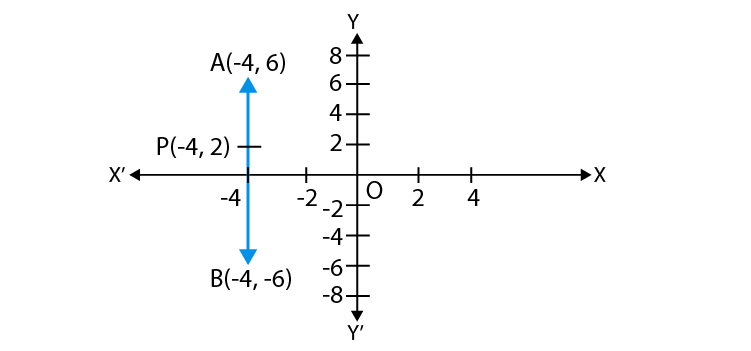Hence, from the graph it is clear that, point P ( – 4, 2) lies on the line segment joining the points A ( – 4, 6) and B ( – 4, – 6),

3. The points (0, 5), (0, –9) and (3, 6) are collinear.

Solution:

False

Justification:

The points are collinear if area of a triangle formed by its points is equals to the zero.

Given,

x1 = 0, x2 = 0, x3 = 3 and

y1 = 5, y2 = – 9, y3 = 6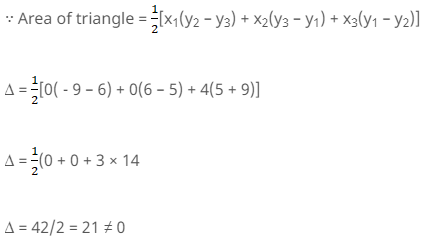From the above equation, it is clear that the points are not collinear.

4. Point P (0, 2) is the point of intersection of y–axis and perpendicular bisector of line segment joining the points A (–1, 1) and B (3, 3).

Solution:

False

Justification:

We know that, the points lying on perpendicular bisector of the line segment joining the two points is equidistant from the two points.

i.e., PA should be equals to the PB.

Using distance formula,

d = √ ((x2 – x1)2 + (y2 – y1)2)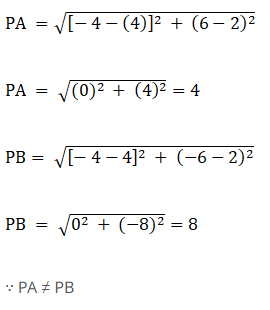5. Points A (3, 1), B (12, –2) and C (0, 2) cannot be the vertices of a triangle.

Solution:

True.

Justification:

Coordinates of A = (x1, y1) = (3, 1)

Coordinates of B = (x2, y2) = (12, – 2)

Coordinates of C = (x3, y3) = (0, 2)

Area of ∆ABC = ∆ = 1/2 [x1 (y2 – y3) + x2 (y3 – y1 ) + x3 (y1 – y2 )]

Δ = ½ [3 – (2 – 2) + 12(2 – 1) + 0{1 – (- 2)}]

Δ = ½ [3(- 4) + 12(1) + 0]

Δ = ½ (- 12 + 12) =0

Area of ΔABC = 0

Since, the points A (3, 1), B (12, – 2) and C (0, 2) are collinear.

Therefore, the points A (3, 1), B (12, – 2) and C (0, 2) can’t be the vertices of a triangle.

6. Points A (4, 3), B (6, 4), C (5, –6) and D (–3, 5) are the vertices of a parallelogram.

Solution:

False

Justification:

The given points are A (4, 3), B (6, 4), C (5, –6) and D (–3, 5)

Finding the distance between A and B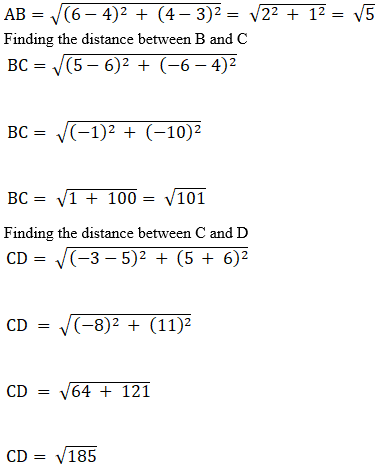Finding the distance between D and A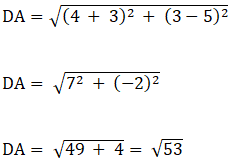Since the distances are different, we can conclude that the points are not the vertices of a parallelogram.

## Exercise 7.3 Page No: 83

1. Name the type of triangle formed by the points A (–5, 6), B (–4, –2) and C (7, 5).

Solution:

The points are A (–5, 6), B (–4, –2) and C (7, 5)

Using distance formula,

d = √ ((x2 – x1)2 + (y2 – y1)2)

AB = √((-4+5)² + (-2-6)²)

= √1+64

=√65

BC=√((7+4)² + (5+2)²)

=√121 + 49

=√170

AC=√((7+5)² + (5-6)²)

=√144 + 1

=√145

Since all sides are of different length, ABC is a scalene triangle.

2. Find the points on the x–axis which are at a distance of 25 from the point (7, –4). How many such points are there?

Solution:

Let coordinates of the point=(x, 0)(given that the point lies on x axis)

x1=7. y1=-4

x2=x. y2=0

Distance =√(x2-x1)2+ (y2-y1)2

According to the question,

2√5=√(x-7)2+ (0-(-4))2

Squaring L.H.S and R.H.S

20=x2+49-14x+16

20=x2+65-14x

0=x2-14x+45

0=x2-9x-5x+45

0=x(x-9)-5(x-9)

0=(x-9) (x-5)

x-9 =0. x-5= 0

x=9 or x=5

Therefore, coordinates of points…..(9,0)or(5,0)

3. What type of a quadrilateral do the points A (2, –2), B (7, 3), C (11, –1) and D (6, –6) taken in that order, form?

Solution:

The points are A (2, –2), B (7, 3), C (11, –1) and D (6, –6)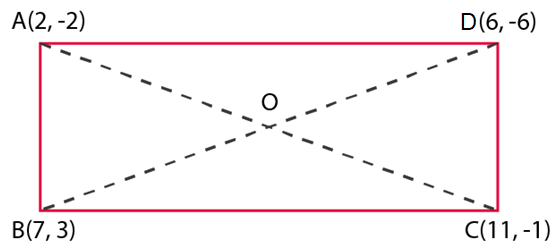Using distance formula,

d = √ ((x2 – x1)2 + (y2 – y1)2)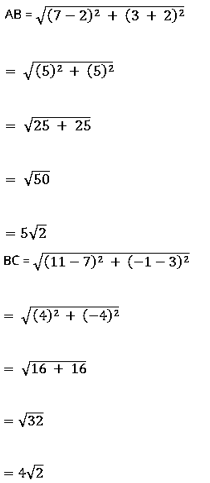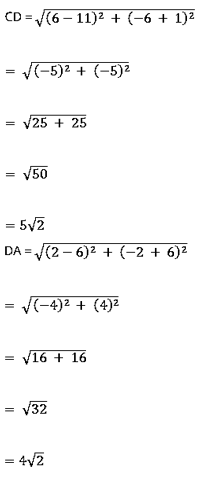Finding diagonals AC and BD, we get,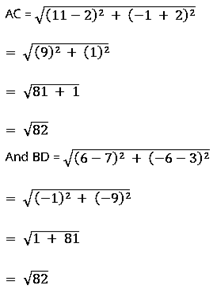4. Find the value of a, if the distance between the points A (–3, –14) and B (a, –5) is 9 units.

Solution:

Distance between two points (x1,y1) ( x2,y2) is :

d=√(x2-x1)²+(y2-y1

Distance between A (–3, –14) and B (a, –5) is :

=√[(a+3)²+(-5+14)²] =9

Squaring on L.H.S and R.H.S.

(a+3)²+81=81

(a+3)²=0

(a+3)(a+3)=0

a+3=0

a= -3

5. Find a point which is equidistant from the points A (–5, 4) and B (–1, 6)? How many such points are there?

Solution:

Let the point be P

According to the question,

P is equidistant from A (–5, 4) and B (–1, 6)

Then the point P = ((x1+x2)/2, (y1+y2)/2)

= ((-5-1)/2, (6+4)/2)

= (-3 , 5 )

6. Find the coordinates of the point Q on the x–axis which lies on the perpendicular bisector of the line segment joining the points A (–5, –2) and B(4, –2). Name the type of triangle formed by the points Q, A and B.

Solution:

Point Q is the midpoint of AB as the point P lies on the perpendicular bisector of AB.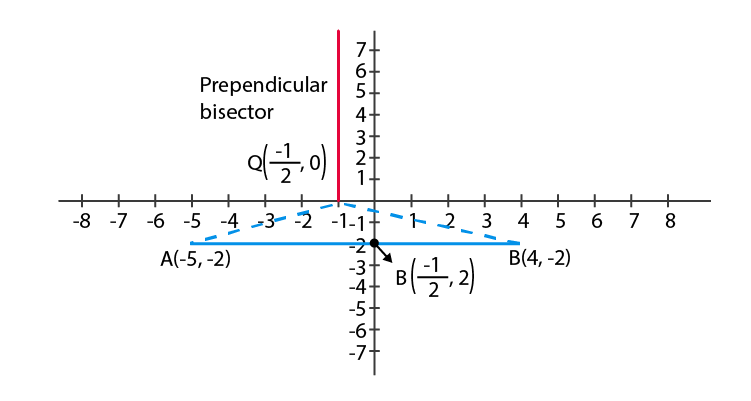By mid point formula:

(x1 + x2)/2 = (-5+4)/2

= -½

x = -½

Given that, P lies on x axis, so y=0

P(x,y)= (-½ , 0)

Therefore, it is an isosceles triangle

7. Find the value of m if the points (5, 1), (–2, –3) and (8, 2m) are collinear.

Solution:

The points A(5, 1), B(–2, –3) and C(8, 2m) are collinear.

i.e., Area of ∆ABC = 0

½ [x1 (y2 – y3 ) + x2 (y3 – y1 ) + x3 (y1 – y2 )]=0

½ [5(-3 – 2m) + ( – 2)(2m – 1) + 8(1 – ( – 3))]=0

½ (-15 – 10m – 4m + 2 + 32) = 0

½ (-14m + 19) = 0

m = 19/14

8. If the point A (2, – 4) is equidistant from P (3, 8) and Q (–10, y), find the values of y. Also find distance PQ.

Solution: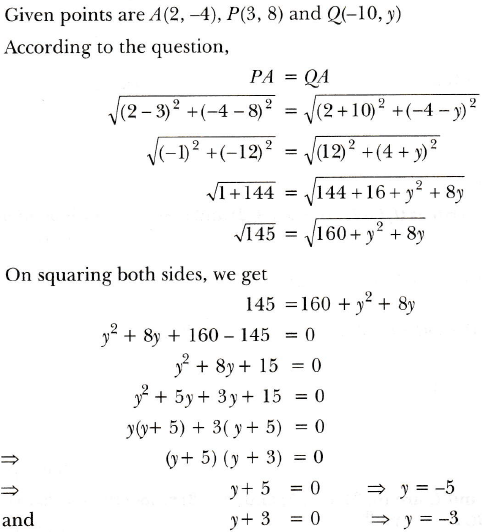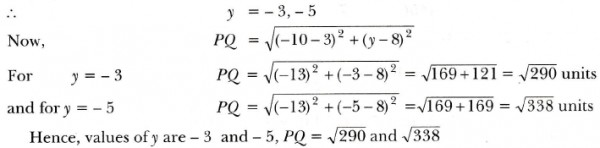9. Find the area of the triangle whose vertices are (–8, 4), (–6, 6) and (–3, 9).

Solution:

Given vertices are:

(x₁, y₁) = (-8, 4)

(x₂, y₂) = (-6, 6)

(x₃, y₃) = (-3, 9)

Area of triangle = (½) (x₁(y₂ – y₃) + x₂(y₃ – y₁) + x₃(y₁ – y₂))

= (½) (-8(6 – 9) + -6(9 – 4) + -3(4 – 6))

= (½) (-8(-3) + -6(5) + -3(-2))

= (½) (24 – 30 + 6)

= (½) (30 – 30)

= 0 units.

10. In what ratio does the x–axis divide the line segment joining the points (– 4, – 6) and (–1, 7)? Find the coordinates of the point of division.

Solution:

Let the ratio in which x-axis divides the line segment joining (–4, –6) and (–1, 7) = 1: k.

Then,

x-coordinate becomes (-1 – 4k) / (k + 1)

y-coordinate becomes (7 – 6k) / (k + 1)

Since P lies on x-axis, y coordinate = 0

(7 – 6k) / (k + 1) = 0

7 – 6k = 0

k = 6/7

Now, m1 = 6 and m2 = 7

By using section formula,

x = (m1x2 + m2x1)/(m1 + m2)

= (6(-1) + 7(-4))/(6+7)

= (-6-28)/13

= -34/13

So, now

y = (6(7) + 7(-6))/(6+7)

= (42-42)/13

= 0

Hence, the coordinates of P are (-34/13, 0)

## Exercise 7.4 Page No: 85

1. If (– 4, 3) and (4, 3) are two vertices of an equilateral triangle, find the coordinates of the third vertex, given that the origin lies in the interior of the triangle.

Solution: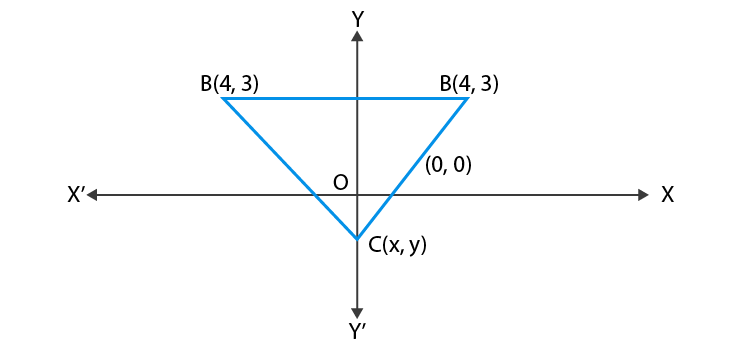Let the vertices be (x,y)

Distance between (x,y) & (4,3) is = √((x-4)2 + (y-3)2)……(1)

Distance between (x,y) & (-4,3) is = √((x+4)2 + (y-3)2)……(2)

Distance between (4,3) &(-4,3) is =√((4+4)2 + (3-3)2) = √(8)²=8

According to the question,

Equation (1)=(2)

(x-4)²=(x+4)²

x²-8x+16=x²+8x+16

16x=0

x=0

Also, equation (1)=8

(x-4)²+(y-3)²=64……… (3)

Substituting the value of x in (3)

Then (0-4)²+(y-3)²=64

(y-3)²=64-16

(y-3)²=48

y-3=(+)4√3

y=3(+) 4√3

Neglect y = 3+4√3 as if y = 3+4√3 then origin cannot interior of triangle

Therefore, the third vertex = (0, 3-4√3)

2. A (6, 1), B (8, 2) and C (9, 4) are three vertices of a parallelogram ABCD. If E is the midpoint of DC, find the area of ADE.

Solution:

According to the question,

The three vertices of a parallelogram ABCD are A (6, 1), B (8, 2) and C (9, 4)

Let the fourth vertex of parallelogram = (x, y),

We know that, diagonals of a parallelogram bisect each other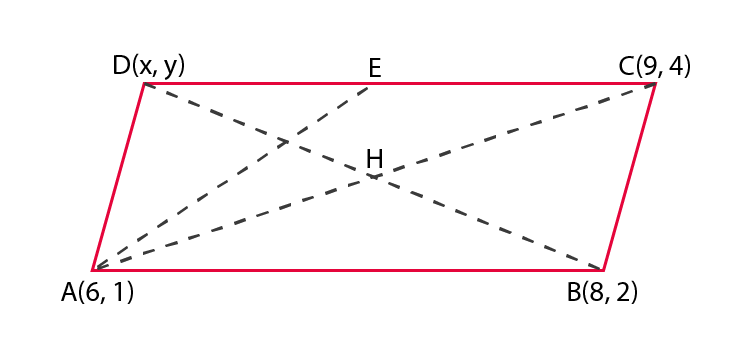Since, mid – point of a line segment joining the points (x1, y1) and (x2, y2) is given by,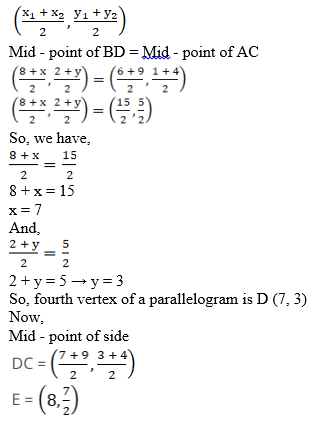∵ Area of ΔABC with vertices (x1, y1), (x2, y2) and (x3, y3);

= ½[x1(y2 – y3) + x2(y3 – y1) + x3(y1 – y2)]

∴ Area of ΔADE with vertices A (6, 1), D (7, 3) and E (8, (7/2))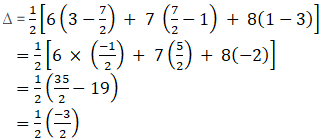= – ¾ but area can’t be negative

Hence, the required area of ΔADE is ¾ sq. units

3. The points A (x1, y1), B (x2, y2) and C (x3 y3) are the vertices of ABC.

(i) The median from A meets BC at D. Find the coordinates of the point D.

(ii) Find the coordinates of the point P on AD such that AP : PD = 2 : 1

(iii) Find the coordinates of points Q and R on medians BE and CF, respectively such that BQ : QE = 2 : 1 and CR : RF = 2 : 1

(iv) What are the coordinates of the centroid of the triangle ABC?

Solution:

According to the question,

The vertices of ΔABC = A, B and C

Coordinates of A, B and C = A(x1, y1), B(x2, y2), C(x3, y3)

(i) As per given information D is the mid – point of BC and it bisect the line into two equal parts.

Coordinates of the mid – point of BC;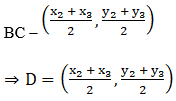(ii) Let the coordinates of a point P be (x, y)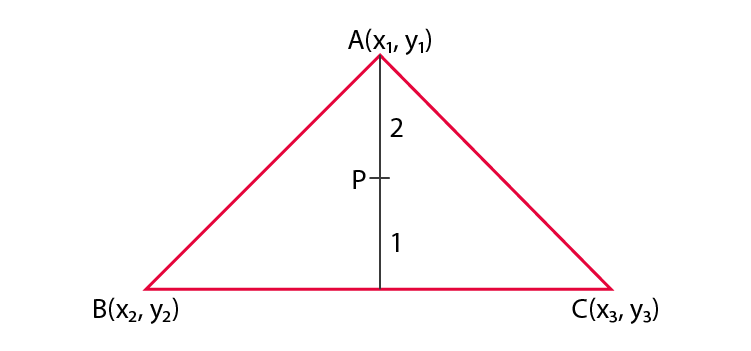Given,

The ratio in which the point P(x, y), divide the line joining,

A(x1, y1) and D((x2+x3)/2 , (y2+y3)/2)) = 2:1

Then,

Coordinates of P =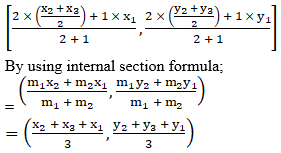(iii) Let the coordinates of a point Q be (p, q)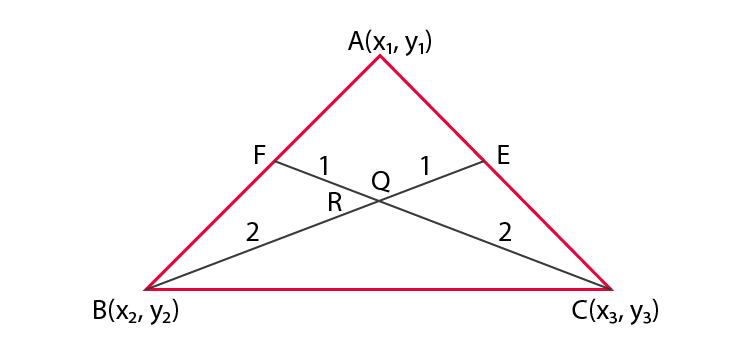Given,

The point Q (p, q),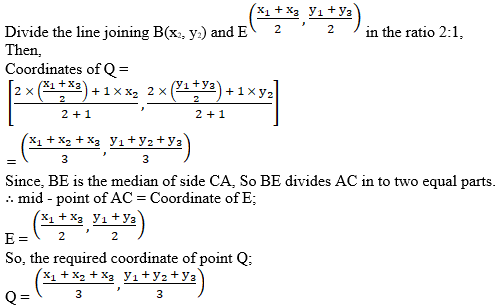Now,

Let the coordinates of a point E be (⍺, β)

Given,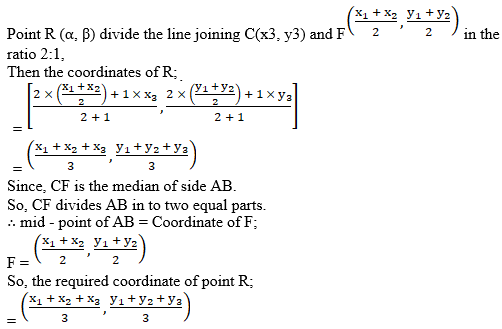(iv) Coordinate of the centroid of the ΔABC;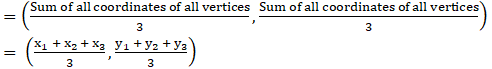Students can freely download PDF of NCERT Exemplar for Chapter 7 – Coordinate Geometry to increase their understanding of all the concepts and practice problems in a more efficient manner. Click here to solve exemplars for all the chapters of Maths Class 10.

Class 10 students are advised to solve sample papers and previous year question papers to have an idea of the type of questions asked from chapter Coordinate Geometry. They can also refer to our online learning materials such as notes, exemplar books, Maths NCERT Solutions for Class 10, and question papers to have a quick revision before the exam.

Download BYJU’S app where you will get personalized video lesson explaining different types of Maths concepts in an easy to understand way.

## Frequently Asked Questions on NCERT Exemplar Solutions for Class 10 Maths Chapter 7

### What is the meaning of coordinate geometry in NCERT Exemplar Solutions for Class 10 Maths Chapter 7?

Coordinate Geometry is considered to be one of the most interesting concepts of mathematics. Coordinate Geometry (or the analytic geometry) describes the link between geometry and algebra through graphs involving curves and lines. It provides geometric aspects in Algebra and enables them to solve geometric problems. It is a part of geometry where the position of points on the plane is described using an ordered pair of numbers. This chapter is also considered to be one of the important chapters from the exam point of view.

### Is Chapter 7 of NCERT Exemplar Solutions for Class 10 Maths difficult to understand?

No, Chapter 7 of NCERT Exemplar Solutions for Class 10 Maths is not difficult to understand. It is one of the interesting topics in Class 10 which would be continued in higher levels of education also. Good knowledge of integral formulas will make it easier for the students to solve the basic sums of integration efficiently. A proper knowledge of coordinate geometry is necessary to find the distance between two points, dividing lines in m:n ratio, finding the midpoint of a line, calculating the area of a triangle in the Cartesian plane, etc.

### Where can I download the NCERT Exemplar Solutions for Class 10 Maths Chapter 7 PDF online?

You can find the NCERT Exemplar Solutions for Class 10 Maths Chapter 7 on BYJU’S. The solutions are prepared by the highly experienced faculty having vast conceptual knowledge. These are considered to be one of the best rated solutions available online. All the problems from the NCERT textbook are solved in a step wise manner based on the latest syllabus and exam pattern designed by the CBSE board.